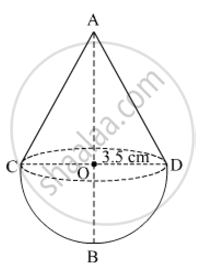# A toy is in the form of a cone of base radius 3.5 cm mounted on a hemisphere of base diameter 7 cm. If the total height of the toy is 15.5 cm, find the total surface area of the top (Use π = 22/7) - Mathematics

A toy is in the form of a cone of base radius 3.5 cm mounted on a hemisphere of base diameter 7 cm. If the total height of the toy is 15.5 cm, find the total surface area of the top (Use π = 22/7)

#### SolutionLet r and h be the radius and height of the cone mounted on the hemisphere, respectively.

Suppose R be the radius of the hemishpere.

Now,

r = R = 3.5 cm

Height of the cone + Radius of the hemisphere = Total height of the toy

h + 3.5 cm = 15.5 cm

h = 15.5 − 3.5 = 12 cm

Let l be the slant height of the cone.

l2=r2+h2

=>l^2=(7/2)^2+(12)^2=49/4+144=625/4

=>l = 25/2cm

Total surface area of the toy

= Curved surface area of the cone + Curved surface area of the hemisphere

=πrl+2πr2

=πr(l+2r)

=22/7xx7/2xx(25/2+2xx7/2)

=22/7xx7/2xx39/2

=214.5 cm2

Concept: Surface Area of a Combination of Solids
Is there an error in this question or solution?